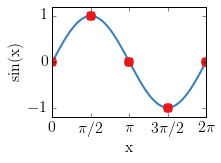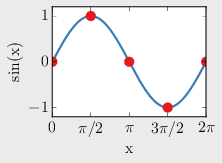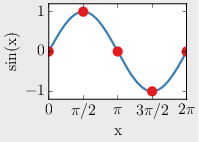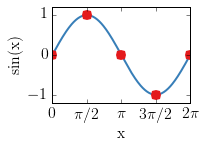# Check the dimension of a pdf-figure

#### 30 September 2014

I like consistency, and I like it when the LaTeX text and the included figures appear as one entity. To achieve this you want to set the font in the figure to the same font as in the text, or at least use the same font for every figure; the same applies to the font size. Furthermore, I want the line thickness to be the same in all figures, no matter how big or small the figure is. The colours should be consistent, the dashes, and so on. I used little scripts to set my matplotlibrc to achieve this. By doing some research for this blog entry I found out that Matplotlib introduces stylesheets with version 1.4, which makes using your own style, or different styles, very easy:

>>> from matplotlib import style
>>> style.use('yourstyle')
>>> print(plt.style.available)


What a great feature! Use the latter to see which pre-defined styles are available.

There was one thing though that kept bugging me, and that is what I talk about in this fifth post of the aftershock series. I tend to save Matplotlib figures as pdf to include them in the LaTeX document. And I want to include them without specifying width nor height, for the same reason as mentioned above: I want the font size and line width to be consistent, which is not the case if you scale your image. However, this requires that the pdf you want to include has the correct dimension. That is where checksize comes in handy. To use checksize you need to install PyPDF2. I also installed wand, to plot pdf’s in the notebook for the examples; it is not required for checksize.

(ipynb)$pip install PyPDF2, wand  ## checksize() The function checksize saves a figure as a pdf with plt.savefig. It then reads the created pdf with PyPDF2 and compares the pdf-size with the specified, desired size. If the difference is bigger than the defined precision, it adjusts the figure-size and calls recursively checksize again, until the precision is matched. In : import numpy as np import matplotlib.pyplot as plt from PyPDF2 import PdfFileReader # Read pdf from wand.image import Image as Image # Plot pdf # Increase font size, set CM as default text, and use LaTeX rc('font', **{'size': 16, 'family': 'serif', 'serif': ['Computer Modern Roman']}) rc('text', usetex=True) # Define colours (taken from http://colorbrewer2.org) clr = ['#377eb8', '#e41a1c', '#4daf4a', '#984ea3', '#ff7f00', '#ffff33', '#a65628']  ### Load the checksize-function (You can find it in the notebook adashof.ipynb, in the same repo as this notebook). In : %load -s checksize adashof.py  In : def checksize(fhndl, name, dsize, precision=0.01, extent=0.05, kwargs={}, _cf=False): """Print figure with 'name.pdf', check size, compare with dsize, and adjust if required Parameters ---------- fhndl : figure-handle Figure handle of the figure to be saved. name : string Figure name. dsize : list of two floats Desired size of pdf in cm. precision : float, optional; <0.01> Desired precision in cm of the dimension, defaults to 1 mm. extent : float or list of floats, optional; <0.01> - If float, then bbox_inches is set to tight, and pad_inches=extent. - If it is an array of two numbers it sets the percentaged extent-width, Bbox.expanded. - If it is an array of four numbers it sets [x0, y0, x1, y1] of Bbox. kwargs : dict Other input arguments that will be passed on to plt.savefig; e.g. dpi or facecolor. _cf : Internal parameter for recursion and adjustment. """ # Import PyPDF2 from PyPDF2 import PdfFileReader # Check extent input and set bbox_inches and pad_inches accordingly if np.size(extent) == 1: bbox_inches = 'tight' pad_inches = extent else: fext = fhndl.gca().get_window_extent().transformed( fhndl.dpi_scale_trans.inverted()) if np.size(extent) == 2: bbox_inches = fext.expanded(extent, extent) elif np.size(extent) == 4: fext.x0, fext.y0, fext.x1, fext.y1 = extent extent = [1, 1] # set extent to [1, 1] for recursion bbox_inches = fext pad_inches=0 # Save the figure fhndl.savefig(name+'.pdf', bbox_inches=bbox_inches, pad_inches=pad_inches, **kwargs) # Get pdf-dimensions in cm pdffile = PdfFileReader(open(name+'.pdf', mode='rb')) pdfsize = np.array([float(pdffile.getPage(0).mediaBox), float(pdffile.getPage(0).mediaBox)]) pdfdim = pdfsize*2.54/72. # points to cm # Define print-precision on desired precision pprec = abs(int(('%.1e' % precision).split('e')))+1 # Get difference btw desired and actual size diff = dsize-pdfdim # If diff>precision, adjust, else finish if np.any(abs(diff) > precision): if not _cf: _cf = [1, 1] # Be verbose print(' resize...') # Adjust width if (abs(diff) > precision): print(' X-diff:', np.round(diff, pprec), 'cm') # Set new factor to old factor times (desired size)/(actual size) _cf = _cf*dsize/pdfdim # Set new figure width fhndl.set_figwidth(_cf*dsize/2.54) # cm2in # Adjust height if (abs(diff) > precision): print(' Y-diff:', np.round(diff, pprec), 'cm') # Set new factor to old factor times (desired size)/(actual size) _cf = _cf*dsize/pdfdim # Set new figure height fhndl.set_figheight(_cf*dsize/2.54) #cm2in # Call the function again, with new factor _cf figsize = checksize(fhndl, name, dsize, precision, extent, kwargs, _cf) return figsize else: # Print some info if the desired dimensions are reached # Print figure name and pdf dimensions print('Figure saved to '+name +'.pdf;', np.round(pdfdim, pprec), 'x', np.round(pdfdim, pprec), 'cm.') # Print the new figsize if it had to be adjusted if _cf: print(' => NEW FIG-SIZE: figsize=('+ str(np.round(fhndl.get_size_inches(), 2*pprec))+', '+ str(np.round(fhndl.get_size_inches(), 2*pprec))+')') # Return figsize return fhndl.get_size_inches()  ### Example Generate some data to plot. In : xdata = np.linspace(0, 2*np.pi, 201) ydata = np.sin(xdata) xpdata = np.arange(5)*np.pi/2 ypdata = np.sin(xpdata) # Small function to plot the data def plot_data(): plt.plot(xdata, ydata, c=clr, lw=2) plt.plot(xpdata, ypdata, 'o', mec='none', mfc=clr, ms=10) plt.xlabel('x') plt.ylabel('sin(x)') plt.xticks(xpdata, (r'0', r'$\pi$/2', r'$\pi$', r'3$\pi$/2', r'2$\pi\$'))
plt.yticks([-1, 0, 1])
plt.axis([0, 2*np.pi, -1.2, 1.2])

# Small function to load pdf and get dimension
def get_pdf_dim(name):
pdfsize = np.array([float(pdffile.getPage(0).mediaBox),
float(pdffile.getPage(0).mediaBox)])
return np.round(pdfsize*2.54/72., 3) # points to cm, rounded


First I create a normal plot. I would like a plot of dimensions 7 cm by 5 cm. The figsize argument of plt.figure takes inches, hence I have to divide our centimetres by 2.54.

In :
fig1 = plt.figure(figsize=(7/2.54, 5/2.54))
plot_data()I now save the figure with plt.savefig, with a facecolor in order to see exactly what was saved if I load the figure again. If I check the dimensions of the pdf, we see that the actual size is bigger than the specified size of 7 cm by 5 cm. The actual size depends on the tick-labels, on the axis-labels, titles, and other factors.

In :
# Save the figure to 'orig.pdf'
fig1.savefig('data/checksize/orig.pdf', bbox_inches='tight', facecolor='.9')

# Get pdf-dimensions
pdfdim = get_pdf_dim('data/checksize/orig.pdf')
print('Pdf width x height:', pdfdim, 'x', pdfdim, 'cm')

Image(filename='data/checksize/orig.pdf')

Pdf width x height: 7.819 x 5.763 cm


Out:I save the figure now with checksize, providing the desired dimension of 7 cm by 5 cm; I do not specify the precision, the default precision of 1 mm is therefore applied.

In :
figsize = checksize(fig1, 'data/checksize/check1', dsize=[7.0, 5.0], kwargs={'facecolor':'.9'})

  resize...
X-diff: -0.565 cm
Y-diff: -0.509 cm
resize...
X-diff: -0.16 cm
Y-diff: -0.151 cm
resize...
X-diff: -0.048 cm
Y-diff: -0.048 cm
resize...
X-diff: -0.015 cm
Y-diff: -0.015 cm
Figure saved to data/checksize/check1.pdf; 7.004 x 5.005 cm.
=> NEW FIG-SIZE: figsize=(2.470962, 1.712652)



checksize detects that the output dimension is different from the desired dimension, and adjusts it accordingly. If the precision is set too low, this process can take many iterations, and even fall into and endless loop. But one millimetre or a tenth of it is generally not a problem.

To check if checksize did a proper job, I do the same to control the pdf dimension as I did before:

In :
# Get pdf-dimensions
pdfdim = get_pdf_dim('data/checksize/check1.pdf')
print('Pdf width x height:', pdfdim, 'x', pdfdim, 'cm')

Image(filename='data/checksize/check1.pdf')

Pdf width x height: 7.004 x 5.005 cm


Out:If checksize has to change the dimensions, it prints the new figure size at the end, and also returns the new values. I can now provide the updated figsize when I create the figure:

In :
fig2 = plt.figure(figsize=figsize)
plot_data()
figsize = checksize(fig2, 'data/checksize/check2', dsize=[7.0, 5.0], kwargs={'facecolor':'.9'})

Figure saved to data/checksize/check2.pdf; 7.004 x 5.005 cm.With the adjusted figsize the figure is saved with the correct dimensions straight away, no looping is required anymore. Of course, it is likely to change if you make changes to the plot.

You can play around with the extent argument to adjust the space around the figure. For some figures, checksize does not work very well; e.g. figures with 3D axis, or when the axis are set to equal. Again, have a go with the extent-parameter, it might help in those cases too.

You can find the above notebook Checksize.ipynb on my GitHub page in the blog-notebooks-repo.

## Decay

This fifth post concludes the series, the aftershocks decayed.

However, should you ever have a question regarding my thesis, either with respect to geophysics, Python, or LaTeX, please drop me a line, and I will see what I can do.